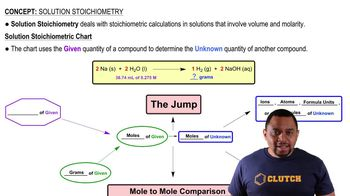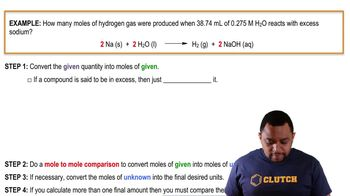Start typing, then use the up and down arrows to select an option from the list.
12:09 minutes
Problem 92
Textbook Question

# The following three solutions are mixed: 100.0 mL of 0.100 M Na2SO4, 50.0 mL of 0.300 M ZnCl2, and 100.0 mL of 0.200 M Ba(CN)2. (b) What is the molarity of each ion remaining in the solution assuming complete precipitation of all insoluble compounds?Verified Solution
This video solution was recommended by our tutors as helpful for the problem above.
96views